Courses

# Application of the First Law to Closed Systems Civil Engineering (CE) Notes | EduRev

## Civil Engineering (CE) : Application of the First Law to Closed Systems Civil Engineering (CE) Notes | EduRev

The document Application of the First Law to Closed Systems Civil Engineering (CE) Notes | EduRev is a part of the Civil Engineering (CE) Course Thermodynamics.
All you need of Civil Engineering (CE) at this link: Civil Engineering (CE)

Application of the First Law to Closed Systems

In general, a thermodynamic system in its most complex form may be multi-component as well as multiphase in nature, and may contain species which react chemically with each other. Thermodynamic analysis tends to focus dominantly on the energy changes occurring within such a thermodynamic system due to change of state (or vice versa), and therefore it is often convenient to formulate the first law specifically for the system in question. Here we focus on closed systems, i.e., one that does not allow transfer of mass across its boundary. As already

pointed out work and heat may enter or leave such a system across its boundary (to and fro with respect to the surrounding) and also be stored in the common form of internal energy. Since in a system may also possess potential and kinetic energies, one may reframe the first law as follows.
Using the notations , , for specific internal, kinetic and potential energies, respectively: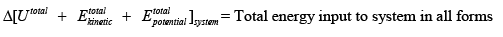If the energy transfer across the system boundary takes place only the form of work and heat: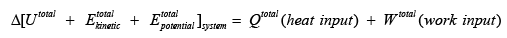The above relation may be written per unit mass / mole of closed system, i.e.,: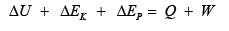The above equation may also be written in a differential form:

dU + dE + dE= δ Q +δ W

If there is no change in potential and kinetic energies for the system or it is negligible – as is usually true for most thermodynamic systems of practical interest – the above equation reduces to:

dU = δ Q +δ W

One of the great strengths of the mathematical statement of the first law as codified by eqn. 3.2 is that it equates a state variable (U) with two path variables (Q, W). As a differentiator we use the symbol δ to indicate infinitesimal work and heat transfer (as opposed to d used state variables).  The last equation potentially allows the calculation of work and heat energies required for a process, by simply computing the change in internal energy. As we shall see later (chapters. 4 & 5) changes in internal energy can be conveniently expressed as functions of changes in state properties such as T, P and V.

In the above equation the term δW represents any form of work transfer to or from the system. In many situations of practical interest the thermodynamic work for closed systems is typically the PdV work (eqn. 1.6). Hence in such cases one may reframe eqn. 3.2 as follows: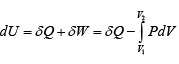In keeping with the definition of work above, we adopt the following convention:

W > 0, If work is done on system

W < 0, If work is done by system

Q > 0,  If work is done to system

Q < 0,If work is done from system

The process of change in a thermodynamic system may occur under various types of constraints, which are enlisted below:

• Constant pressure (isobaric)

• Constant volume (isochoric)

• Constant temperature (isothermal)

The mathematical treatment of each of these processes is presented below.
For a constant pressure process (fig. 3.2), we may write: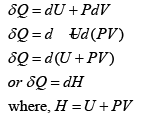The term H is termed enthalpy. It follows that like U, H is also a state variable.
On integrating the differential form of the equation above one obtains for the process: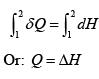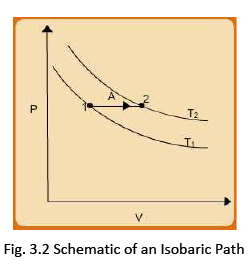On the other hand if the process occurs under isochoric (const. V) conditions (shown in fig. 3.3) the first law leads to: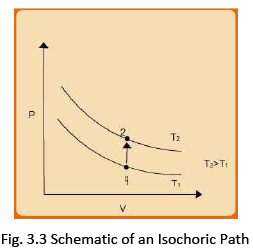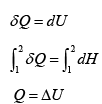We have already seen that a body can retain heat in the form of internal energy. This gives rise
to the concept of heat capacity C and is mathematically defined as: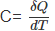It follows that using eqns. 3.4 and 3.5 two types of heat capacity may be defined:

• Constant pressure heat capacity  Csuch that: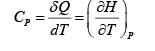.....(3.6)

• Constant pressure heat capacity  Cv such that: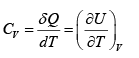.....(3.7)

Thus, using eqns. (3.6) and (3.7) one may rewrite eqns. (3.4) and (3.5) as follows: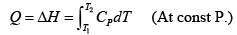.....(3.8)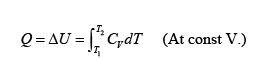.....(3.9)

With the addition of heat to a system, the translation, vibrational and rotational (as well as subatomic) energies of the molecules are enhanced and so it may be expected that the specific heats would be dependent on temperature. On the other hand when substances are compressed intermolecular interactions begin to contribute to internal energy (as hence to enthalpy) and therefore specific heats are rendered pressure dependent. For the case of ideal gases, however, the specific heats are independent of pressure as there are no intermolecular interactions; they are only temperature dependent. Values of specific heats of ideal gases (ig), say at constant pressure, are available from experimental measurements and are typically expressed in the form of polynomials such as: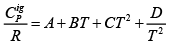Where, A, B, C and D are characteristic constants for a substance, and R is the universal gas constant. Values of the constants in eqn. (3.10) are readily available for a large number of pure substances (see Appendix III for values of select gases). Fig. 3.4 shows typical dependence of const, pressure specific heat for select substances with temperature.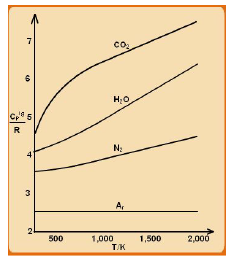Fig. 3.4 Variation of C P ig/R vs. Temperature (Source: J. M Smith, H.C. Van Ness, M.M. Abbott, Introduction to Chemical Engineering Thermodynamics, 6th ed., McGraw-Hill, 2001) Values of the coefficients of similar specific heat capacity polynomials for liquids and solids are available elsewhere (see for example, J.M. Smith, H.C. Van Ness and M.M. Abbott, Introduction to Chemical Engineering Thermodynamics, 6th ed., McGraw-Hill, 2001).

Applications to Ideal Gases

The other two types of thermodynamic processes – isothermal and adiabatic – in closed systems are conveniently understood by applying the first law to a system comprised of an ideal gas. For such a case the relationship between U and H may be rewritten using the EOS:
H = U + PV = U + RT
Or: H − U= RT

Since both H and U are only temperature dependent for ideal gases we write: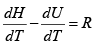Now using eqns. 3.6 and 3.7 it follows that: PV
Cp−Cv= R                .....(3.11)

(It may be noted that since the molar volume V is relatively small for both liquids and solids, one may write: H ≈U ; hence: CP=CV ).

Let us now consider the relationships that obtain for an isothermal process for an ideal gas. Using eqn. 3.2, sincedU= 0:

δ Q = −δW
Or, Q = – W

If we consider only P-V work, the work term is calculable if the process is carried out reversibly, as the ideal gas EOS relate the P and V at all points of change, hence: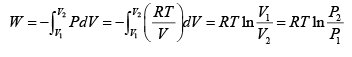.....(3.12)

Thus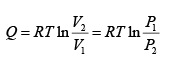.....(3.13)

For isobaric process: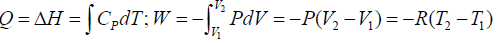...(3.14)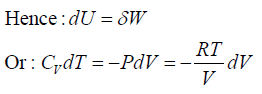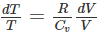...(3.15)

The ratio of heat capacities is defined as:

γ= Cp/Cv

or: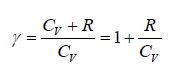...(3.16)

On integrating eqn. 3.15 and using the relationship provided by eqn. 3.16, the following set of results may be derived easily: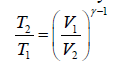............(3.17)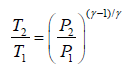......(3.18)

and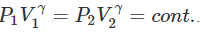.............. (3.19)

Further: dW=dU=CvdT

or W=dU=CvdT

Since,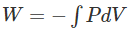Using eqn. 3.19 in the expression for work in the last equation: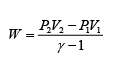.....(3.20)

or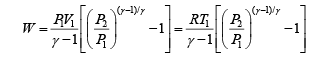.....(3.21)

All the above processes discussed can be captured in the form of a single P-V relation, which is termed a polytropic equation as it can be reduced to yield all forms of processes. The polytropic relations are written by generalizing eqns. 3.17 – 3.19, as follows:

PV δ = constant                .....(3.22)

TV δ −1 = constant                .....(3.23)

TP (1−δ ) /δ = constant ..                .....(3.24)

The schematic of polytropic process is shown in fig. 3.5.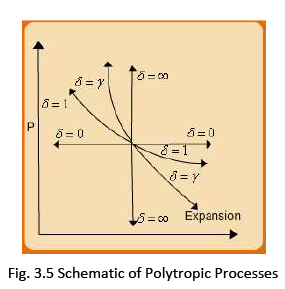As may be seen the various values of δ reproduce the isothermal, isochoric, isobaric and adiabatic processes. In line with eqn. 3.21, for such the generalized expressions for work and heat transfer may be shown to be: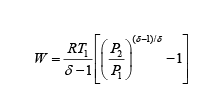....(3.25)

Further: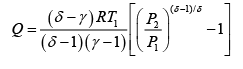....(3.26)

Offer running on EduRev: Apply code STAYHOME200 to get INR 200 off on our premium plan EduRev Infinity!

## Thermodynamics

56 videos|92 docs|33 tests

,

,

,

,

,

,

,

,

,

,

,

,

,

,

,

,

,

,

,

,

,

;